# planeWaveExcitation

Create plane wave excitation environment for antenna or array

## Description

The `planeWaveExcitation` object creates an environment where a plane wave excites an antenna or array. Plane wave excitation is a scattering solution that solves the receiving antenna problem. By default, the antenna element is a dipole. The dipole is excited using a plane wave that travels along the positive x-axis having a z-polarization.

## Creation

### Syntax

``h = planeWaveExcitation``
``h = planeWaveExcitation(Name,Value)``

### Description

example

``` `h = planeWaveExcitation` creates an environment where a plane wave excites the antenna or array. By default, the plane wave excites a dipole antenna.```

example

``` `h = planeWaveExcitation(Name,Value)` returns a `planeWaveExcitation` environment, with additional properties specified by one or more name-value pair arguments. `Name` is the property name and `Value` is the corresponding value. You can specify several name-value pair arguments in any order as `Name1`, `Value1`, `...`, `NameN`, `ValueN`. Properties not specified retain their default values.```

## Properties

expand all

Antenna or array element, specified as an object handle.

Note

For infinite array, support for unit cell analysis is for only transmit scenarios.

Example: `'Element',linearArray`

Incidence of plane wave, specified as a three-element real vector.

Example: `'Direction',[0 0 1]`

Data Types: `double`

Polarization of incident electric field in x, y, and z components, specified as a three-element complex vector in V/m The polarization vector gives the orientation and magnitude of the electric field.

Example: `'Polarization',[0 1 0]`

Data Types: `double`

## Object Functions

 `axialRatio` Axial ratio of antenna `beamwidth` Beamwidth of antenna `charge` Charge distribution on metal or dielectric antenna or array surface `current` Current distribution on metal or dielectric antenna or array surface `EHfields` Electric and magnetic fields of antennas; Embedded electric and magnetic fields of antenna element in arrays `mesh` Mesh properties of metal or dielectric antenna or array structure `meshconfig` Change mesh mode of antenna structure `pattern` Radiation pattern and phase of antenna or array; Embedded pattern of antenna element in array `patternAzimuth` Azimuth pattern of antenna or array `patternElevation` Elevation pattern of antenna or array `show` Display antenna or array structure; display shape as filled patch

## Examples

collapse all

Excite a dipole antenna using a plane wave and view it.

```h = planeWaveExcitation; show(h)```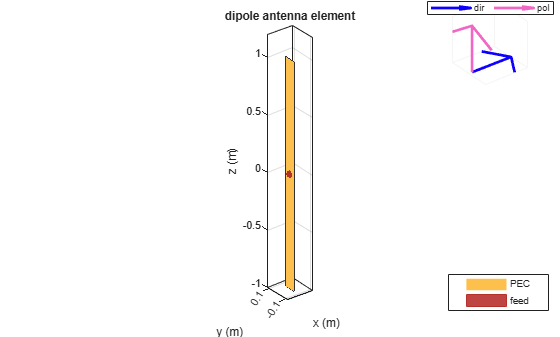The blue arrow shows the direction of propagation of the plane wave. By default, the direction is along the x-axis. The pink arrow shows polarization of the plane wave. By default, the polarization is perpendicular to the direction of propagation i.e. along the z-axis.

Excite a dipole antenna using plane wave. Calculate the feed current at 70 MHz.

```h = planeWaveExcitation cur = feedCurrent(h, 70e6) ```
```h = planeWaveExcitation with properties: Element: [1×1 dipole] Direction: [1 0 0] Polarization: [0 0 1] cur = 0.0179 - 0.0040i ```

Excite a dipole antenna using a plane wave. The polarization of the wave is along the z-axis and the direction of propagation is along the negative x-axis. View the antenna.

```p = planeWaveExcitation('Element', dipole, 'Direction', [-1 0 0], 'Polarization', [0 0 1]); show(p);```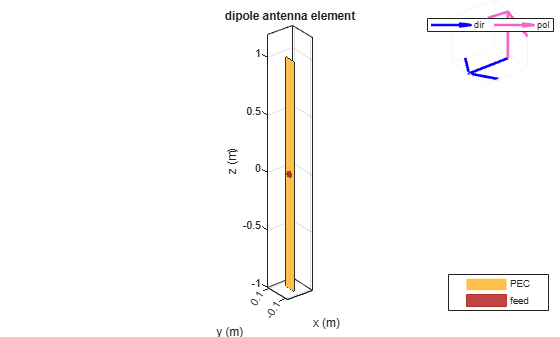Plot the current distribution on the dipole antenna at 70 MHz.

`current(p, 70e6);`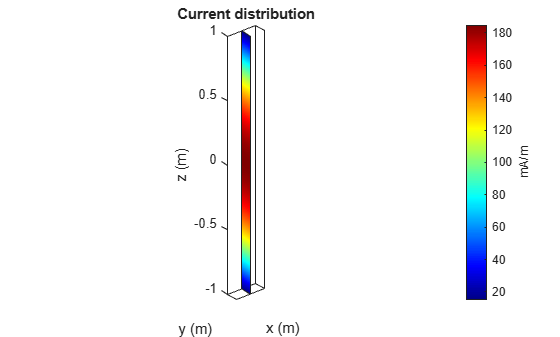Consider a dipole excited by a plane wave.

```p = planeWaveExcitation; p.Direction = [0 1 1]; show(p)```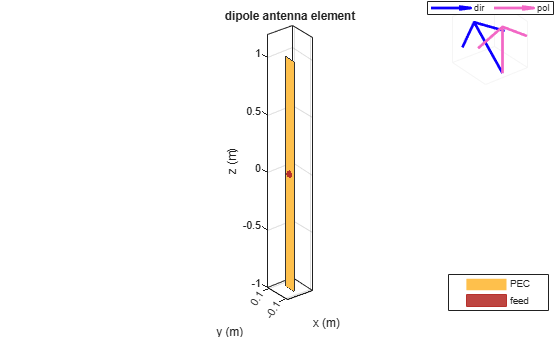If you use the above option, any analysis of this antenna will error out as the polarization and direction vector are not orthogonal to each other.

Use the cross-product function to find the appropriate polarization direction of such a wave.

```p = planeWaveExcitation; p.Polarization = cross(p.Direction, [0 1 1]); show(p);```Calculate the current distribution of the antenna.

`current(p,75e6);`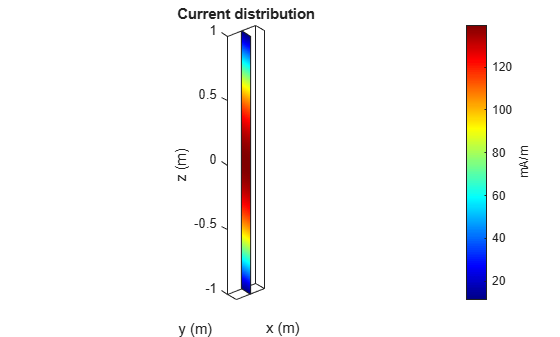Excite an infinite array using a plane wave.

`p = planeWaveExcitation('Element',infiniteArray)`
```p = planeWaveExcitation with properties: Element: [1x1 infiniteArray] Direction: [1 0 0] Polarization: [0 0 1] ```
`show(p)`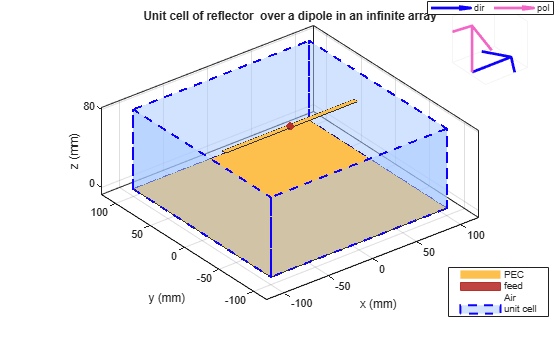Balanis, C. A. Antenna Theory. Analysis and Design. 3rd Ed. Hoboken, NJ: John Wiley & Sons, 2005.